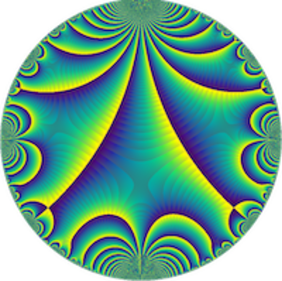# Generalized visible points in random walk paths on Z^k — Kui Liu (Qingdao University)

Séminaire « Arithmétique »
M2 Kampé de Fériet

A lattice point $P\in\mathbb{Z}^k$ $(k\geq 2)$ is said to be visible if there is no other lattice point lying on the line segment joining $P$ and the origin. We study the distribution of generalized visible points (along curves) in random walk paths on $\mathbb{Z}^k$. This a joint work with Meijie Lu and Xianchang Meng.